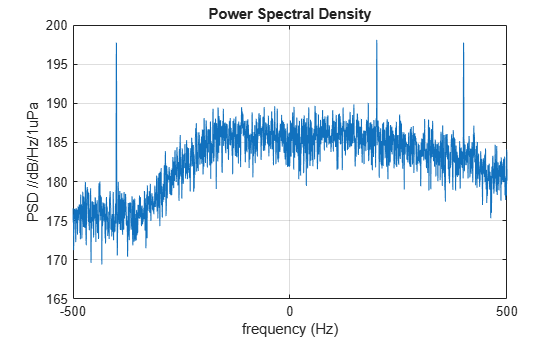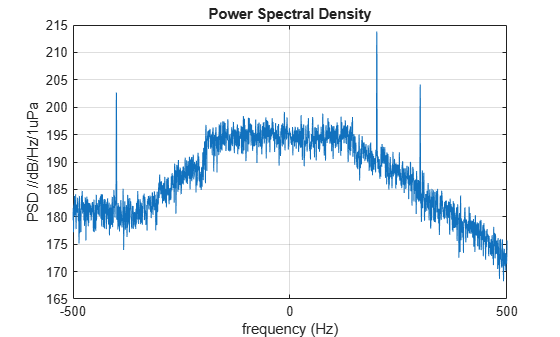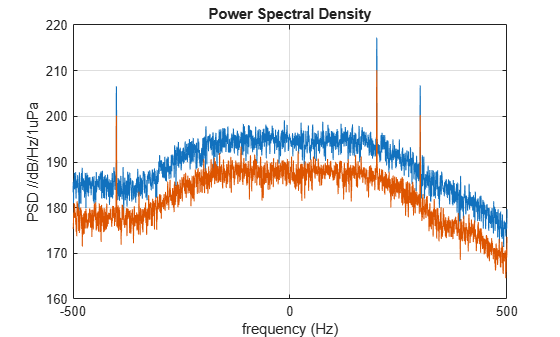Documentation

# phased.UnderwaterRadiatedNoise

Radiate acoustic noise from underwater or surface sound source

## Description

The phased.UnderwaterRadiatedNoise System object™ creates a source of underwater radiated acoustic noise. The noise source can either be on the sea surface or underwater. The radiated noise consists of two components: broadband noise and tonal noise. Broadband noise fills the entire operating system bandwidth while tonal noise occurs at discrete frequencies within the bandwidth. In general, the intensity of the radiated noise depends on the noise spectrum and the source radiation pattern. The object lets you specify

• The spectral shape and levels of the broadband noise.

• The frequencies and levels of the tones.

• The noise source radiation pattern.

To propagate noise from a source to a receiver, use this object with the `phased.IsoSpeedUnderwaterPaths` and the `phased.MultipathChannel` objects.

To generate radiated underwater noise:

1. Create the `phased.UnderwaterRadiatedNoise` object and set its properties.

2. Call the object with arguments, as if it were a function.

To learn more about how System objects work, see What Are System Objects? (MATLAB).

## Creation

### Syntax

``noiseradiator = phased.UnderwaterRadiatedNoise``
``noiseradiator = phased.UnderwaterRadiatedNoise(Name,Value)``

### Description

````noiseradiator = phased.UnderwaterRadiatedNoise` creates an underwater radiated noise source with default property values.```
````noiseradiator = phased.UnderwaterRadiatedNoise(Name,Value)` creates an underwater radiated noise source with each property `Name` set to a specified `Value`. You can specify additional name-value pair arguments in any order as (`Name1`,`Value1`,...,`NameN`,`ValueN`). Enclose each property name in single quotes.Example: ```noiseradiator = phased.UnderwaterRadiatedNoise('TonalLevels',[4700 4900 5150],'SampleRate',500,'OperatingFrequency',5000)``` creates a noise source with tones at 4.7, 4.9, and 5.15 kHz. The sample rate is set to 0.5 kHz and the operating frequency is 5 kHz. The broadband noise levels are set to default values.```

## Properties

expand all

Unless otherwise indicated, properties are nontunable, which means you cannot change their values after calling the object. Objects lock when you call them, and the `release` function unlocks them.

If a property is tunable, you can change its value at any time.

For more information on changing property values, see System Design in MATLAB Using System Objects (MATLAB).

Number of output noise samples, specified as a positive integer.

Example: `500`

Data Types: `double`

Sample rate, specified as a positive scalar. The sample rate together with the operating frequency determines the operating frequency band. See Input and Output Frequency Bands for the definition of the operating frequency band. Units are in Hz.

Example: `2.0e3`

Data Types: `double`

Signal operating frequency, specified as a positive scalar. The operating frequency determines the center of the operating frequency band. See Input and Output Frequency Bands for the definition of the operating frequency band. Units are in Hz.

Example: `15.0e3`

Data Types: `double`

Radiated tonal frequencies, specified as a vector of nonnegative values. Tonal frequencies must lie in the operating frequency band. Tonal frequencies outside this band are ignored. The length of the `TonalFrequencies` vector must match the length of the `TonalLevels` vector. Units are in Hz. See Input and Output Frequency Bands for the definition of the operating frequency band.

Example: `[14900 15010 15200]`

Data Types: `double`

Radiated tonal noise levels, specified as a vector of positive values. Units are in dB//1μPa. The length of the `TonalLevels` vector must match the length of the `TonalFrequencies` vector.

Example: `[50 20 170]`

Data Types: `double`

Broadband noise spectrum level, specified as a vector of real-values. This vector specifies the noise spectrum at uniformly spaced frequencies in the operating system band. Units are in dB/Hz//1μPa.

Example: `[140 145 145 130]`

Data Types: `double`

Azimuth angles of source radiation pattern entries, specified as a length-P vector. This property specifies the azimuth angles of the columns of the source radiation pattern, DirectionalPattern property. P must be greater than 2. Units are in degrees.

Example: `[140 145 145 130]`

Data Types: `double`

Elevation angles of the source radiation pattern entries, specified as a length-Q vector. This property specifies the elevation angles of the rows of the source radiation pattern, DirectionalPattern. Q must be greater than 2. Units are in degrees.

Example: `[-45 -30 0 45 30]`

Data Types: `double`

Source radiation pattern, specified as a real-valued matrix or array. Units are in dB. The allowable pattern dimensions are:

Radiation Pattern Dimensions

DimensionsApplication
Q-by-P matrixSpecifies a directional pattern as a function of Q elevation angles and P azimuth angles. The same pattern is used for all frequencies.
Q-by-P-by-K arraySpecifies a directional pattern as a function of Q elevation angles, P azimuth angles, and K frequencies. If K = 1, the directional pattern is equivalent to a Q-by-P matrix.
1-by-P-by-K arraySpecifies a directional pattern as a function of P azimuth angles and K frequencies. These dimensions apply when there is only one elevation angle.
K-by-P matrix

• Q is the length of the vector specified by the ElevationAngles property.

• P is the length of the vector specified by the AzimuthAngles property.

• K is the number of frequencies specified by the FrequencyVector property.

Matrix and Array Specifications

ApplicationRadiation Pattern Dimensions
One source and M radiation directions specified in the `ang` argument of the object function.Specify one radiation pattern matrix or array for all radiating angles.
M sources with the same pattern and M radiation directions specified in the `ang` argument of the object function.Specify one radiation pattern matrix or array for all radiating angles.
M sources with individual radiation patterns and M radiation directions specified in the `ang` argument of the object function.M radiation patterns in a cell array. All patterns must have the same sizes and types. The number of patterns must match the number of radiating angles.

Example: `[1,3;5,-10]`

Data Types: `double`

Radiation pattern frequencies, specified as a positive, real-valued 1-by-K vector. The vector defines the frequencies at which the DirectionalPattern property values are specified. The elements of the vector must be in strictly increasing order and frequencies must lie in the operating frequency band. See Input and Output Frequency Bands for the definition of the operating frequency band. Units are in Hz.

Example: `1e6`

Data Types: `double`

Random number generator seed source, specified as `'Auto'` or `'Property'`. The random numbers are used to generate the noise. When you set this property to `'Auto'`, random numbers are generated using the default MATLAB random number generator. When you set this property to `'Property'`, the object uses a private random number generator with a seed specified by the `Seed` property.

To use this object with Parallel Computing Toolbox software, set this property to `'Auto'`.

Data Types: `char`

Random number generator seed, specified as a nonnegative integer less than 232.

Example: `10223`

#### Dependencies

To enable this property, set the SeedSource property to `'Property'`.

Data Types: `double`

## Usage

### Syntax

``y = radiatednoise(ang)``

### Description

example

````y = radiatednoise(ang)` returns the noise,`y`, radiated in the direction, `ang`.```

### Input Arguments

expand all

Noise radiation directions, specified as a real-valued 2-by-M matrix. Each column of `ang` specifies the direction of radiation of the corresponding noise signal in the form `[AzimuthAngle;ElevationAngle]`. When `ang` represents multiple angles, the DirectionalPattern property can contain one pattern or M patterns. In that case, each column of `ang` corresponds to one of the patterns. If there is only one pattern, then the multiple noise signals are generated using the same source pattern. Units are in degrees.

Example: `[0 20; 35 -10]`

Data Types: `double`

### Output Arguments

expand all

Radiated noise, specified as a complex-valued M-by-N matrix. M is the number of angles specified in the `ang` argument. N is the number of samples specified by the `NumSamples` property. Radiated noise lies in the baseband range [-fs/2 fs/2]. fs represents the sample rate set by the SampleRate property. Noise units are in Pa.

Data Types: `double`
Complex Number Support: Yes

## Object Functions

To use an object function, specify the System object as the first input argument. For example, to release system resources of a System object named `obj`, use this syntax:

`release(obj)`

expand all

 `step` Run System object algorithm `release` Release resources and allow changes to System object property values and input characteristics `reset` Reset internal states of System object

The `reset` object function resets the random number generator state when the SeedSource property is set to` 'Property'`.

## Examples

expand all

Generate radiated noise from a surface ship. The sonar operating frequency is 5.0 kHz and the sampling rate is 1.0 kHz. By definition, broadband noise band lies in the band 4.5 kHz to 5.5 kHz. In addition, there are tonal noises at 4.6, 5.2, and 5.4 kHz.

```shippos = [0;0;0]; rcvpos = [100;0;-50];```

Compute the noise transmission angle from the ship to the receiver.

`[~,ang] = rangeangle(rcvpos,shippos)`
```ang = 2×1 0 -26.5651 ```

Construct a `phased.UnderwaterRadiatedNoise` System object™ having a radiation pattern that depends only on elevation angle. Compute the noise radiated in the direction of the receiver. Create 10000 samples of the noise radiated towards the target.

```azang = [-180:180]; elang = [-80:80]; pattern = mag2db(repmat(cosd(elang)',1,numel(azang))); fs = 1000; noiseradiator = phased.UnderwaterRadiatedNoise('NumSamples',10000, ... 'SampleRate',fs,'TonalFrequencies',[4600 5200 5400],'TonalLevels',[200,200,200], ... 'BroadbandLevels',[180 180 190 190 190 188 185],'AzimuthAngles',azang, ... 'ElevationAngles',elang,'DirectionalPattern',pattern, ... 'OperatingFrequency',5e3,'SeedSource','Property','Seed',2781);```

Generate 10000 samples of noise.

`y = noiseradiator(ang);`

Plot the noise power spectral density (psd). Convert the psd to intensity referenced to 1uPa.

```[psd,fr] = pwelch(y,[],[],[],noiseradiator.SampleRate,'psd','centered'); plot(fr,10*log10(psd*1e12)); title('Power Spectral Density') xlabel('frequency (Hz)') ylabel('PSD //dB/Hz/1uPa') grid```The three tones appear over the broadband spectrum.

Generate radiated noise from an underwater vehicle. Assume that the noise radiation pattern depends on frequency. The sonar operating frequency is 5.0 kHz and the sampling rate is 1.0 kHz. By definition, the broadband noise band is from 4.5 kHz to 5.5 kHz. In addition, there are tonal noises at 4.6, 5.2, and 5.3 kHz. Define the radiation pattern at three frequencies within this band. All three patterns are multiples of the basic pattern. The frequencies of the radiation patterns are 4.6 kHz, 5.0 kHz, and 5.3 kHz.

First, specify the source and receiver positions.

```srcpos = [0;50;-20]; rcvpos = [100;0;-50];```

Compute the noise transmission angle from vehicle to receiver.

`[~,ang] = rangeangle(rcvpos,srcpos)`
```ang = 2×1 -26.5651 -15.0203 ```

Construct a `phased.UnderwaterRadiatedNoise` System object™ with a radiation pattern that depends only the azimuth angle and frequency. Compute the noise radiated in the direction of the receiver. Create 10000 samples of noise radiated from the vehicle.

```azang = [-180:180]; elang = [-90:90]; fc = 5000.0;```

Put the radiation pattern in a three-dimensional array.

```basepattern = repmat(10*cosd(azang).^2,numel(elang),1); pattern(:,:,1) = 0.5*basepattern; pattern(:,:,2) = basepattern; pattern(:,:,3) = 0.6*basepattern; patterndb = mag2db(pattern); noiseradiator = phased.UnderwaterRadiatedNoise('NumSamples',10000, ... 'SampleRate',1e3,'TonalFrequencies',[4600,5200 5300], ... 'TonalLevels',[200,210,200],'BroadbandLevels',[180 180 190 190 190 180 170], ... 'AzimuthAngles',azang,'ElevationAngles',elang, ... 'FrequencyVector',[4600,5000,5300],'DirectionalPattern',pattern, ... 'OperatingFrequency',5e3,'SeedSource','Property','Seed',2081);```

Generate 10000 samples of noise.

`y = noiseradiator(ang);`

Plot the noise power spectral density (psd). Convert the psd to intensity referenced to 1uPa.

```[psd,fr] = pwelch(y,[],[],[],noiseradiator.SampleRate,'psd','centered'); plot(fr,10*log10(psd*1e12)); title('Power Spectral Density') xlabel('frequency (Hz)') ylabel('PSD //dB/Hz/1uPa') grid```The three tones appear over the broadband spectrum.

Generate radiated noise from a two underwater vehicles. Assume that the noise radiation pattern is different for each. The sonar operating frequency is 5.0 kHz and the sampling rate is 1.0 kHz. By definition, the broadband noise band is from 4.5 kHz to 5.5 kHz. In addition, there are tonal noises at 4.6, 5.2, and 5.3 kHz. The frequencies of the radiation patterns are 4.6 kHz, 5.0 kHz, and 5.3 kHz.

First, specify the source and receiver positions.

```srcpos1 = [0;50;-20]; srcpos2 = [200;50;-80]; rcvpos = [100;0;-50];```

Compute the noise transmission angle from vehicle to receiver.

```[~,ang1] = rangeangle(rcvpos,srcpos1); [~,ang2] = rangeangle(rcvpos,srcpos2);```

Construct a `phased.UnderwaterRadiatedNoise` System object™ with a radiation pattern that depends only the azimuth angle and frequency. Compute the noise radiated in the direction of the receiver. Create 10000 samples of noise radiated from the vehicle.

```azang = [-180:180]; elang = [-90:90]; fc = 5000.0;```

Put the radiation pattern in a three-dimensional array.

```pattern1 = repmat(10*cosd(azang).^2,numel(elang),1); pattern2 = ones(181,361); pattern1db = mag2db(pattern1); pattern2db = mag2db(pattern2); noiseradiator = phased.UnderwaterRadiatedNoise('NumSamples',10000, ... 'SampleRate',1e3,'TonalFrequencies',[4600,5200 5300], ... 'TonalLevels',[200,210,200],'BroadbandLevels',[180 180 190 190 190 180 170], ... 'AzimuthAngles',azang,'ElevationAngles',elang, ... 'FrequencyVector',[4600,5000,5300],'DirectionalPattern',{pattern1,pattern2}, ... 'OperatingFrequency',5e3,'SeedSource','Property','Seed',2081);```

Generate 10000 samples of noise.

`y = noiseradiator([ang1,ang2]);`

Plot the noise power spectral density (psd). Convert the psd to intensity referenced to 1uPa.

```[psd,fr] = pwelch(y,[],[],[],noiseradiator.SampleRate,'psd','centered'); plot(fr,10*log10(psd*1e12)); title('Power Spectral Density') xlabel('frequency (Hz)') ylabel('PSD //dB/Hz/1uPa') grid```The three tones appear over the broadband spectrum.

## More About

expand all

 Urick, R.J. Principles of Underwater Sound, 3rd Edition. New York: Peninsula Publishing, 1996.

#### Hybrid Beamforming for Massive MIMO Phased Array Systems

Download the white paper